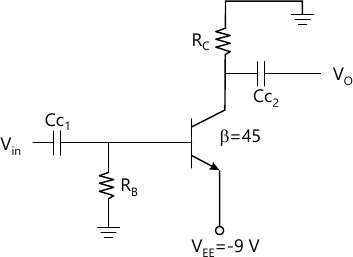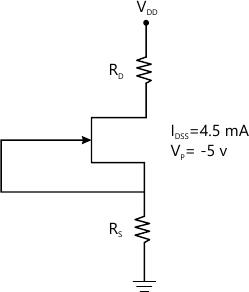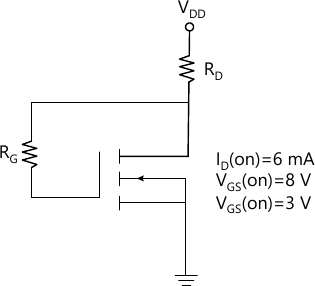MORE IN Analog Electronics - 1
MU Electronics and Telecom Engineering (Semester 3)
Analog Electronics - 1
May 2012
Total marks: --
Total time: --
INSTRUCTIONS
(1) Assume appropriate data and state your reasons
(2) Marks are given to the right of every question
(3) Draw neat diagrams wherever necessary

1 (a) Design single stage R-C coupled CE audio frequency amplifier employing BC147B BJT to satisfy the following requirements. IAvI?100, SICO ?10, Load resistor RL=10k? and output voltage Vo=3volts.
15 M
1 (b) For the designed amplifier in part (a) determine; expected voltage gain, input impedance, output impedance and current supplied by source voltage VCC {Use complete h-model for part(a) and (b) }
5 M

2 (a) Design single stage R-C coupled CS audio frequency amplifier employing JFET BFW-11 to satisfy the following requirements. |Av|?10, VGSO=0.3Vp, RL=120? VDD=20 vlts and output peak voltage V0=4.5volts
12 M
2 (b) For the above designed circuit with source resistor ?RS? unbypassed determine; voltage gain, input impedance, output impedance and output voltage for input voltage of 20 Vpp.
8 M

3 (a) For the circuit shown in Fig A. determine the following: 1. Voltage at collector terminal VC 2. Voltage at base terminal VB 3. Stability SICO 4. Voltage gain10 M
3 (b) For the network of Fig B. With RD=2k2, RS=0.68k? and VDD=20volts determine the following: 1. IDQ and VGSQ 2. VDSQ and VD 3. VG and VS 4. IDQ if RS is bypassed by capacitor CS10 M

Compare the following (Any Four)
4 (a) Output characteristics of CE and CS amplifier
5 M
4 (b) BJT series and BJT shunt voltage regulator
5 M
4 (c) D-MOSFET and E-MOSFET
5 M
4 (d) SCR and TRIAC
5 M
4 (e) LC and C-L-C filter
5 M

5 (a) Design a full wave rectifier dc supply using centre tapped transformer with two diodes to give dc output voltage at 300 volts to a variable resistive load. The load current expected is 75 ± 25 mA with ripple factor not to exceed 0.07. Use C-L-C filter.
12 M
5 (b) For the network of Fig C with RD=2K?, RG=10M?, and VDD=12volts.Determine the following: 1. IDQ 2. VDSQ8 M

6 (a) Describe the operation of BJT series regulator and derive an expression for line regulation(Sv) and load regulation (Ro)
12 M
6 (b) Explain with the help of a circuit diagram the working of a UJT relaxation oscillator.
8 M

Explain the following (any four)
7 (a) Self thermal stability of JFET
5 M
7 (b) Utility of h- parameters
5 M
7 (c) Graphical determination of FET parameters
5 M
7 (d) Features of IGBT
5 M
7 (e) BJT as a Switch
5 M
7 (f) Power MOSFET
5 M

More question papers from Analog Electronics - 1